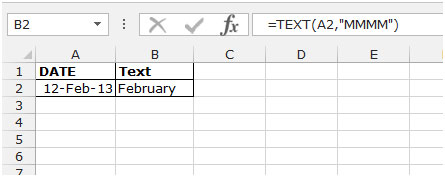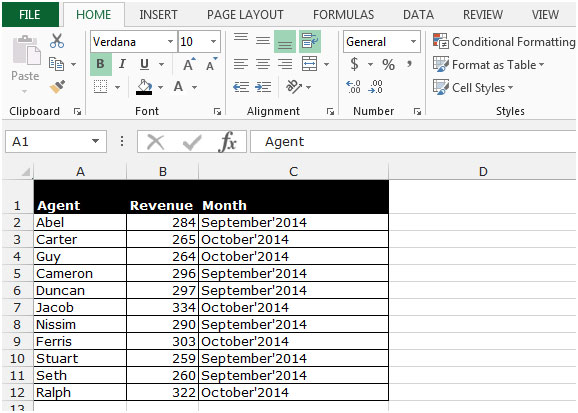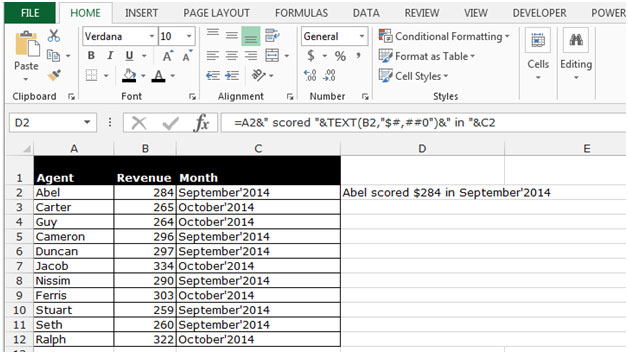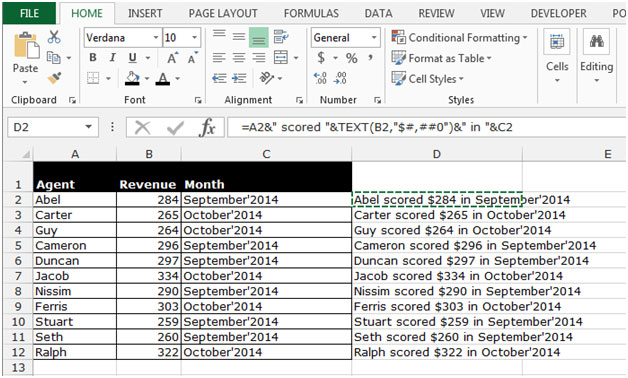# Combining Text and Numerically Calculated Results into One Output String in Microsoft Excel 2010

In this article, we will learn how to join two text in excel and numerically calculated result into one output string. We use the TEXT function in Microsoft Excel 2010 to get the desired output.

TEXT:- This function is used to convert a value to text in a specific number format.

Syntax of “TEXT” function:    =TEXT (Value, format_text)

For Example: -

• Cell B2 contains the date 12-Feb-2013
• Write the formula in cell C2 =TEXT(A2,"MMMM"), and press Enter on your keyboard.
• The function will return the month name.Let’s take an example to understand how we can combine cells in excel and numerically calculated results into one output string.

We want to combine the data from cells A2:C2 into a single cell and ensuring that the number in cell B2 is formatted as currency.

In this article, we use TEXT function to provide the format to number, use the “&” symbol to merge the number and text together.We want to prepare the statement for all agents such as: - Abel scored \$346 in September’2014.

• Write the formula in cell D2.
• =A2&" scored "&TEXT(B2,"\$#,##0")&" in "&C2
• Press Enter on your keyboard.
• The function will combine the text and formatted number.• Copy the same formula by pressing the key Ctrl+C and paste in the range D3:D12 by pressing the key Ctrl+V on your keyboard.This is the way we can combine the text and numerically calculated result into one output string in Microsoft Excel.

## Users are saying about us...

1.thanks

2.If you're having trouble with the formula, make sure to use straight quotes unlike the article which uses left/right double quotes.

C2 =TEXT(A2,”MMMM”)
?
C2 =TEXT(A2,"MMMM")

•That's right.

Terms and Conditions of use

The applications/code on this site are distributed as is and without warranties or liability. In no event shall the owner of the copyrights, or the authors of the applications/code be liable for any loss of profit, any problems or any damage resulting from the use or evaluation of the applications/code.